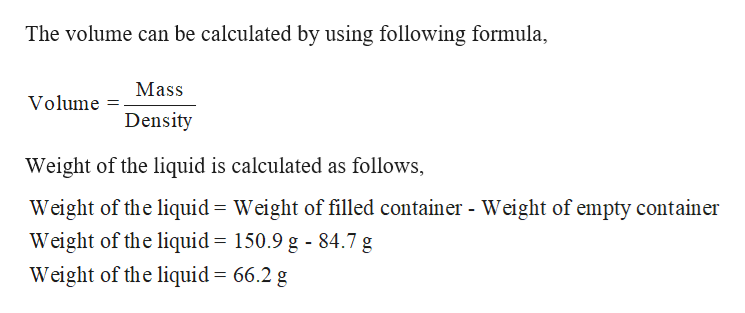# An empty container with an unknown volume is weighed and found to have a mass of 84.7 g. The container is then filled with a liquid with a density of 1.005 g/cm3 and reweighed. The mass of the container and the liquid is 150.9 g. Determine the volume of the container to the appropriate number of significant figures.

Question

An empty container with an unknown volume is weighed and found to have a mass of

84.7 g. The container is then filled with a liquid with a density of 1.005 g/cm3 and reweighed. The mass of the container and the liquid is 150.9 g. Determine the volume of the container to the appropriate number of significant figures.
check_circleExpert Solution
Step 1

Given,

Mass of the container = 84.7 g.

Liquid density = 1.005 g/cm3

M...help_outlineImage TranscriptioncloseThe volume can be calculated by using following formula Mass Volume Density Weight of the liquid is calculated as follows, Weight of the liquid Weight of filled container - Weight of empty container Weight of the liquid = 150.9 g - 84.7 g Weight of the liquid = 66.2 g fullscreen

### Want to see the full answer?

See Solution

#### Want to see this answer and more?

Solutions are written by subject experts who are available 24/7. Questions are typically answered within 1 hour*

See Solution
*Response times may vary by subject and question
Tagged in

### General Chemistry